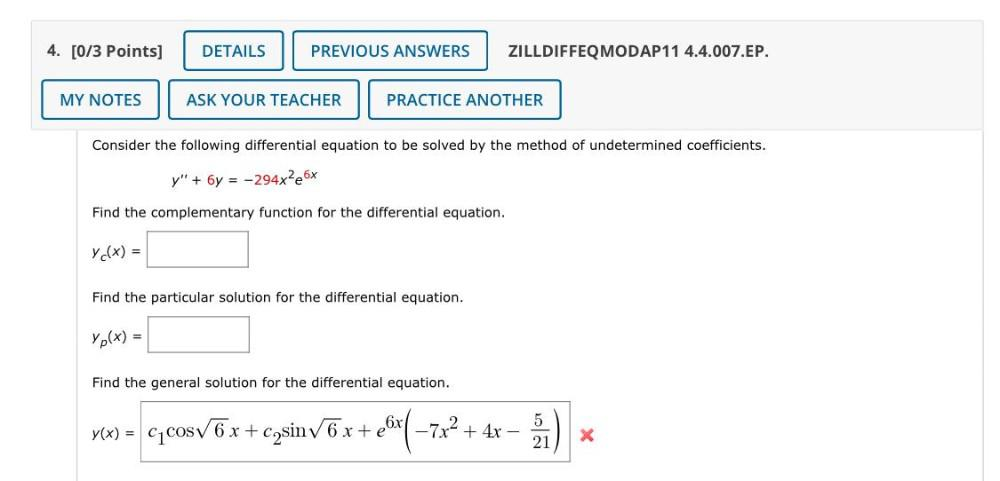Question:

# 4. [0/3 Points] DETAILS PREVIOUS ANSWERS ZILLDIFFEQMODAP11 4.4.007.EP. MY NOTES ASK YOUR TEACHER PRACTICE ANOTHER Consider the f4. [0/3 Points] DETAILS PREVIOUS ANSWERS ZILLDIFFEQMODAP11 4.4.007.EP. MY NOTES ASK YOUR TEACHER PRACTICE ANOTHER Consider the following differential equation to be solved by the method of undetermined coefficients. Y" + 6y = -294xPe6x Find the complementary function for the differential equation. y c(x) = Find the particular solution for the differential equation. Yp(x) = Find the general solution for the differential equation. vlx) = cycosV6x+cosiny 6 x + ell(+7x2 + 4x – ) e 5 21 X